# 坑 6 数据处理

• 一维数据看分布与异常值
• 二维数据看关系与趋势
• 三维看维度间两两关系
• 高维数据降维
• 相似个体聚类与网络结构

## 6.2 统计推断

$\begin{eqnarray*} E\left[\sum_{i=1}^n (X_i - \bar X)^2\right] & = & \sum_{i=1}^n E\left[X_i^2\right] - n E\left[\bar X^2\right] \\ \\ & = & \sum_{i=1}^n \left\{Var(X_i) + \mu^2\right\} - n \left\{Var(\bar X) + \mu^2\right\} \\ \\ & = & \sum_{i=1}^n \left\{\sigma^2 + \mu^2\right\} - n \left\{\sigma^2 / n + \mu^2\right\} \\ \\ & = & n \sigma^2 + n \mu ^ 2 - \sigma^2 - n \mu^2 \\ \\ & = & (n - 1) \sigma^2 \end{eqnarray*}$

$\begin{eqnarray*} Var(\bar X) & = & Var \left( \frac{1}{n}\sum_{i=1}^n X_i \right)\\ \\ & = & \frac{1}{n^2} Var\left(\sum_{i=1}^n X_i \right)\\ \\ & = & \frac{1}{n^2} \sum_{i=1}^n Var(X_i) \\ \\ & = & \frac{1}{n^2} \times n\sigma^2 \\ \\ & = & \frac{\sigma^2}{n} \end{eqnarray*}$

1, 1, 1, 1, 1, 2, 2, 2, 2, 2, 3, 3, 3, 3, 3

# 随机数的10000次比较
set.seed(42)
pvalue <- NULL
for (i in 1:10000){
a <- rnorm(10)
b <- rnorm(10)
c <- t.test(a,b)
pvalue[i] <- c$p.value } # 看下p值分布 hist(pvalue)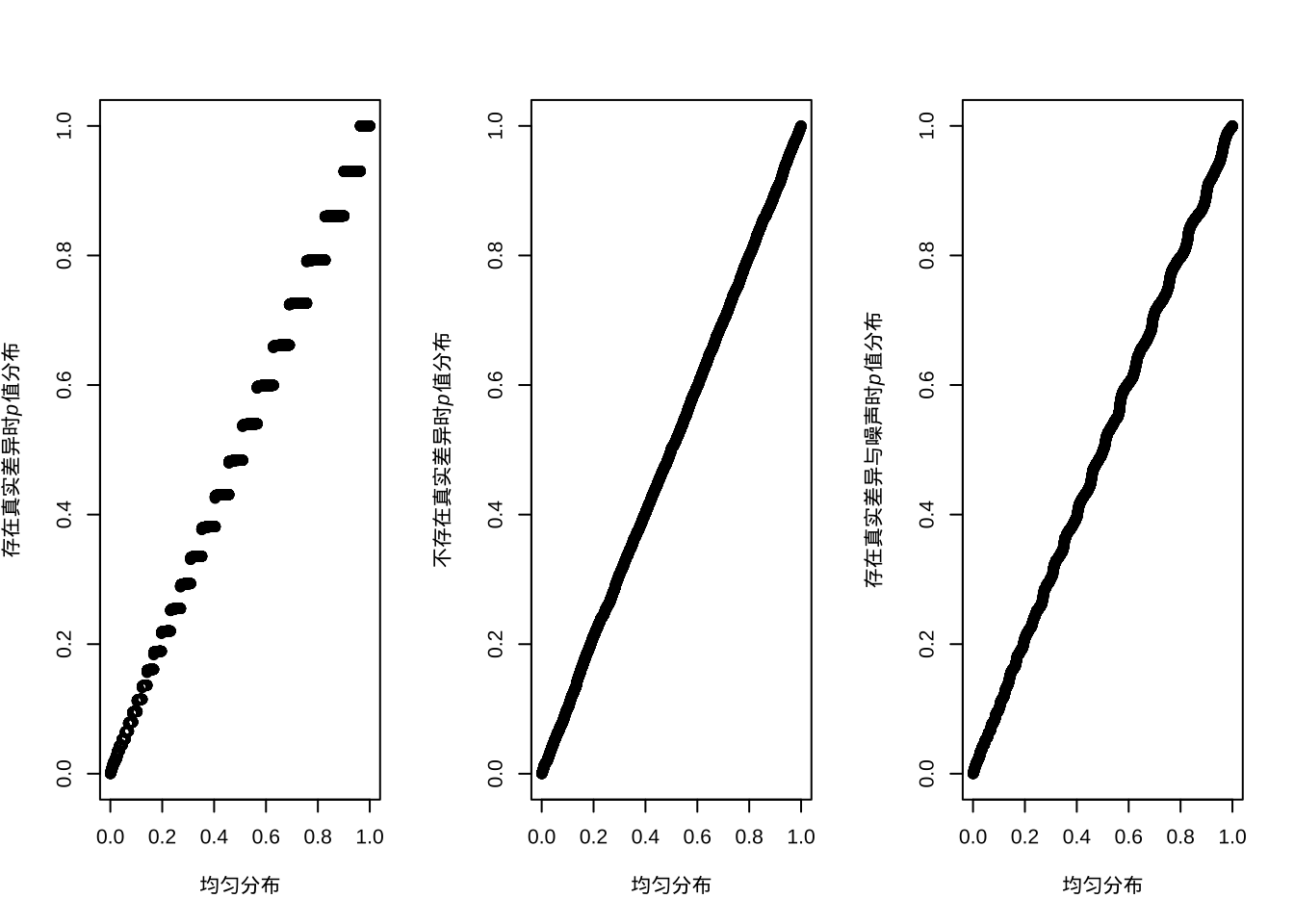# 小于0.05的个数 sum(pvalue<0.05) ##  477 # 小于0.0001的个数 sum(pvalue<0.0001) ##  0 这样我们会看到进行了整体的控制之后，确实是找不到有差异的了，而且此时我们也可以看到p值的分布应该是0到1之间的均匀分布。但假如里面本来就有有差异的呢？ set.seed(42) pvalue <- NULL for (i in 1:10000){ a <- rnorm(10,1) b <- a+1 c <- t.test(a,b) pvalue[i] <- c$p.value
}
# 看下p值分布
hist(pvalue)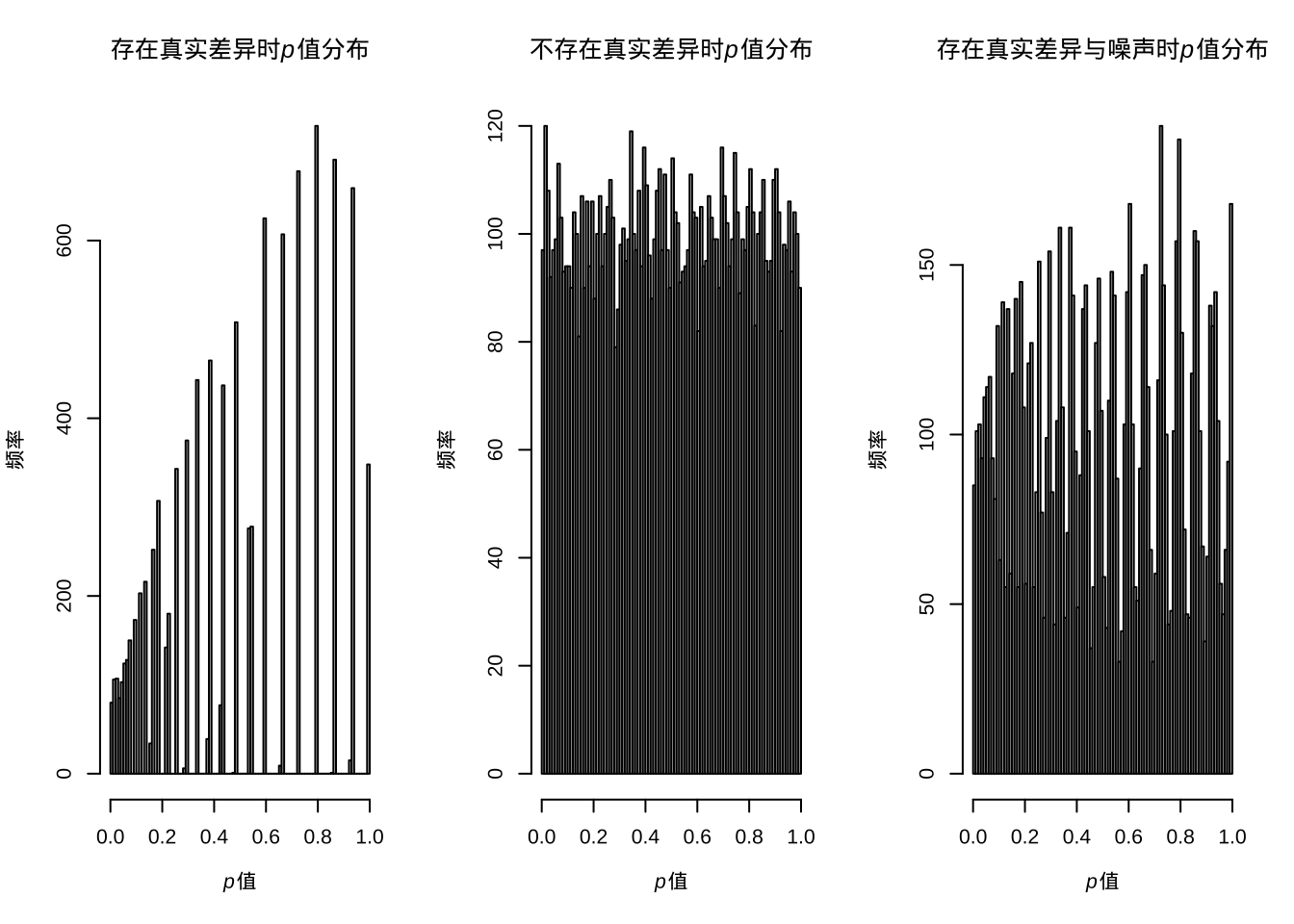# 小于0.05的个数
sum(pvalue<0.05)
##  6559
# 小于0.0001的个数
sum(pvalue<0.0001)
##  45

set.seed(42)
pvalue <- NULL
for (i in 1:5000){
a <- rnorm(10,1)
b <- a+1
c <- t.test(a,b)
pvalue[i] <- c$p.value } for (i in 1:5000){ a <- rnorm(10,1) b <- rnorm(10,1) c <- t.test(a,b) pvalue[i+5000] <- c$p.value
}
# 看下p值分布
hist(pvalue)# 小于0.05的个数
sum(pvalue<0.05)
##  3499
# 小于0.0001的个数
sum(pvalue<0.0001)
##  21

$P(至少一个显著)=1-P(无显著差异) = 1-(1-\alpha/n)^n$

# 比较次数
n <- c(1:10 %o% 10^(1:2))
# 整体出现假阳性概率
p0 <- 1-(1-0.05)^n
# Bonferroni方法控制后的整体假阳性概率
p <- 1-(1-0.05/n)^n
# 不进行控制
plot(p0~n,ylim = c(0,1),xlab='numbers of tests',ylab = 'FWER')
# Bonferroni方法控制
points(p~n,pch=19,col='red')
legend('right',c('Bonferroni','Raw'),col = c('red','black'),pch = 1,cex=1)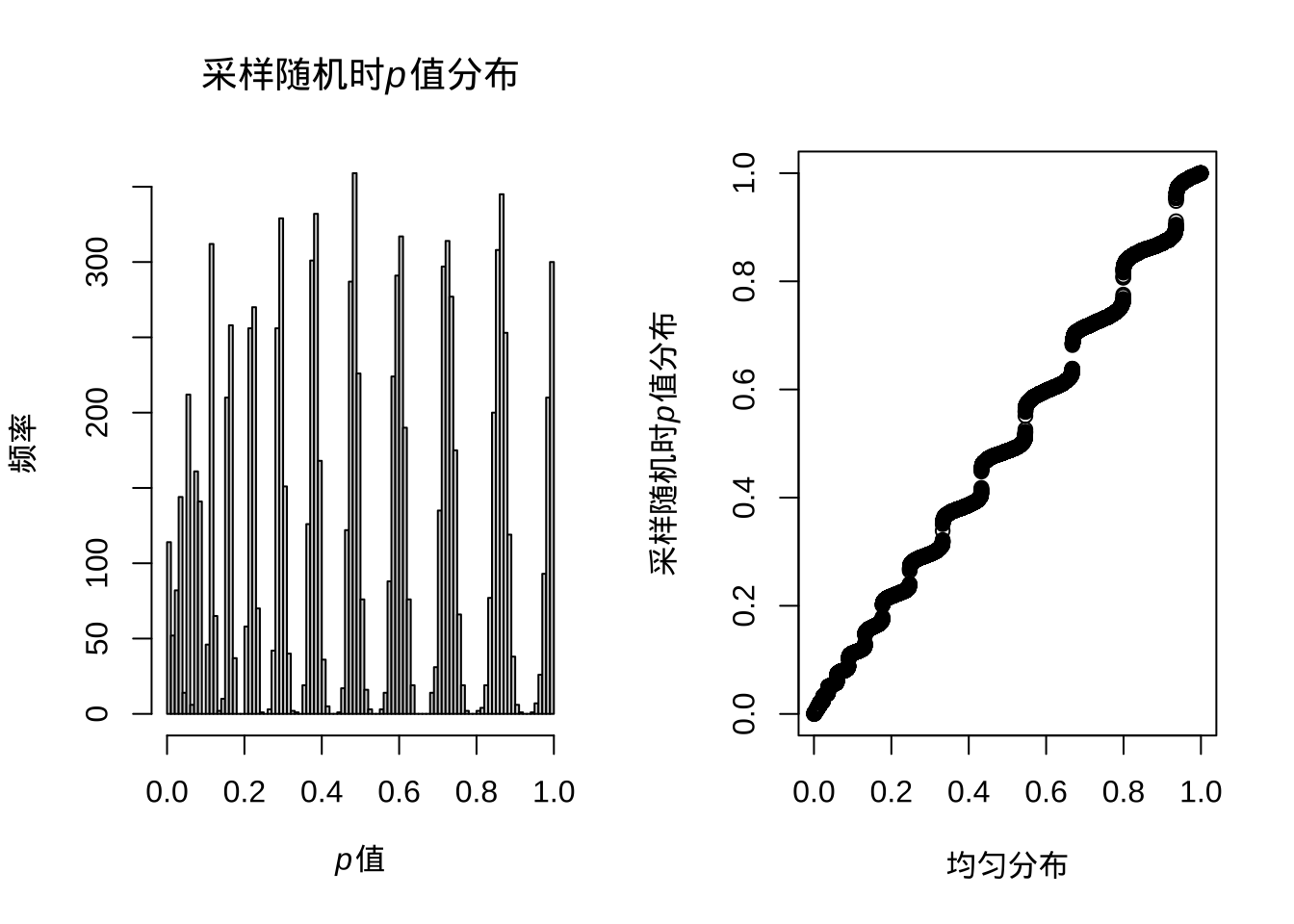$P(至少一个显著)=1-P(无显著差异) = 1-(1-\alpha')^n = 0.05$

# 所有有差异的
R <- sum(pvalue<0.05)
R
##  3499
# 假阳性
V <- sum(pvalue[5001:10000]<0.05)
V
##  225
# 错误发现率
Q <- V/R
Q
##  0.06430409

# 所有有差异的
R <- sum(pvalue<0.01)
R
##  999
# 假阳性
V <- sum(pvalue[5001:10000]<0.01)
V
##  34
# 错误发现率
Q <- V/R
Q
##  0.03403403

Benjamini-Hochberg方法跟Holm方法很像，也是先排序，但之后p值并不是简单的乘排序，而是乘检验总数后除排序：

$p_i \leq \frac{i}{m} \alpha$

# BH法控制错误发现率
pbh <- p.adjust(pvalue,method = 'BH')
# holm法控制错误发现率
ph <- p.adjust(pvalue,method = 'holm')
plot(pbh~pvalue,ylab = 'FDR',xlab = 'p value')
points(ph~pvalue,col='red')
legend('bottomright',c('Holm','BH'),col = c('red','black'),pch = 1,cex=1)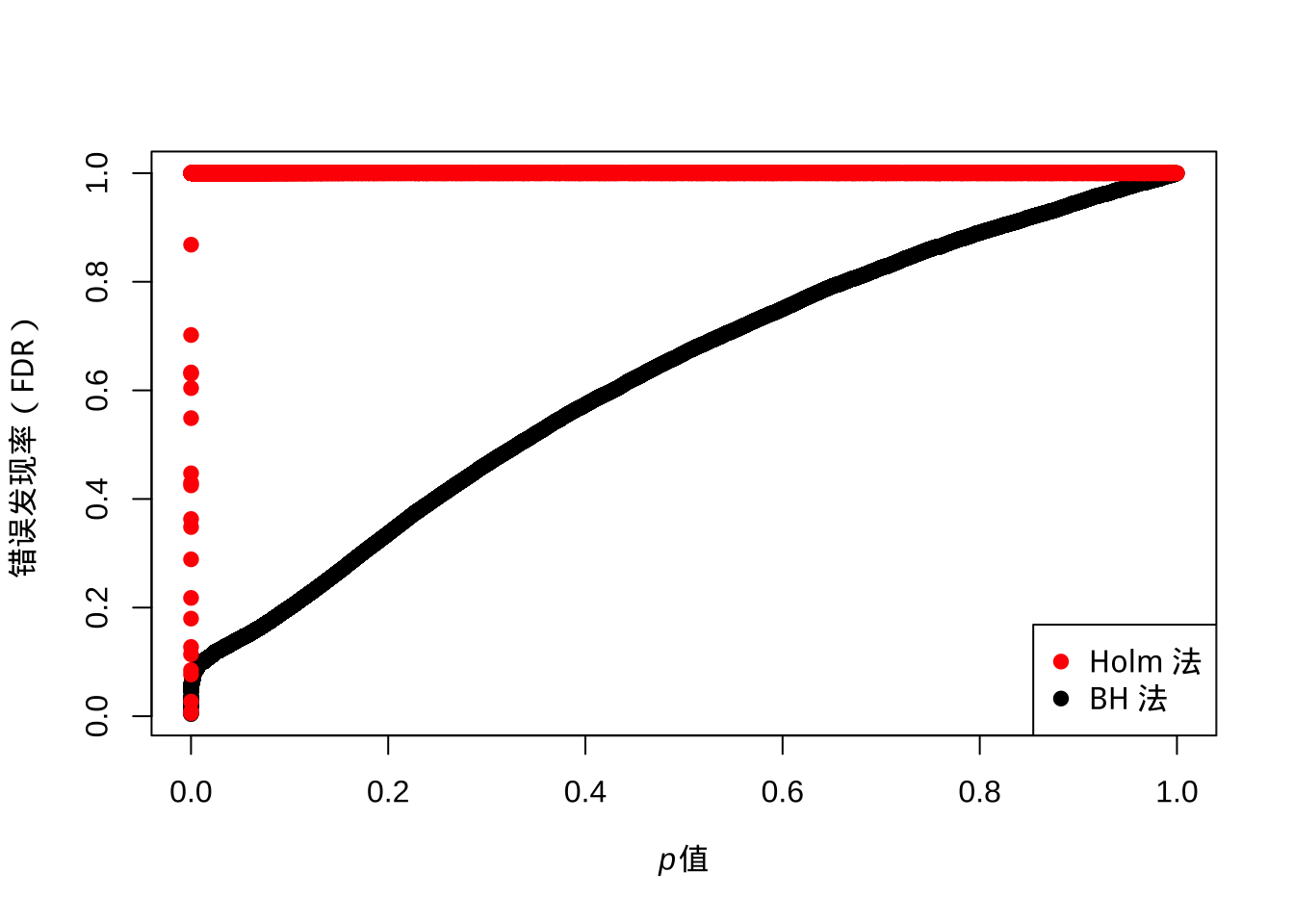$\hat\pi_0 = \frac{\#\{p_i>\lambda\}}{(1-\lambda)m}$

library(qvalue)
q <- qvalue(pvalue)
# Q值
plot(q$qvalues~pvalue,col='blue',ylab = 'Q',xlab = 'p value')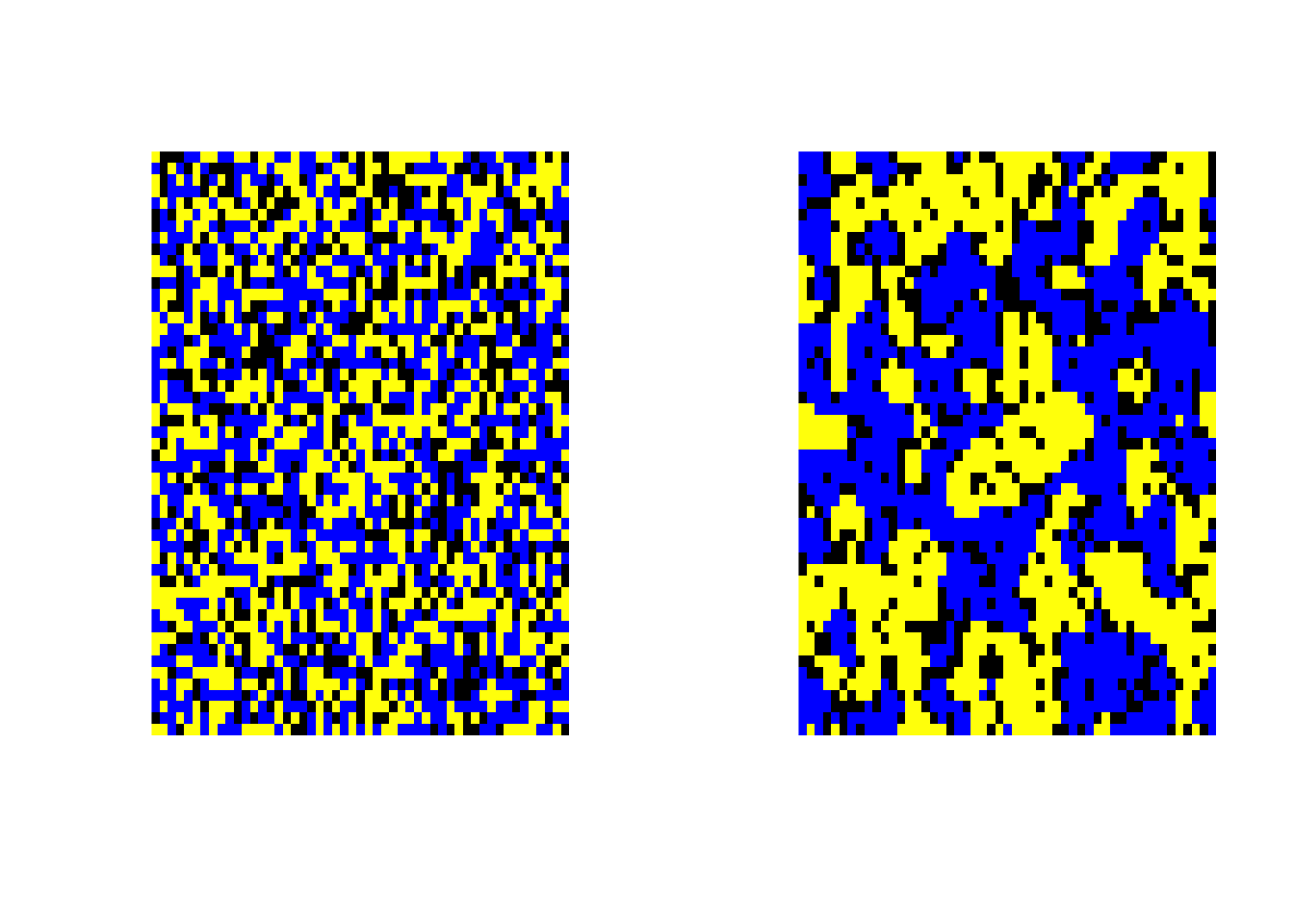如上图所示，q值增大后会最终逼近到0.5，而我们的模拟中零假设的比例就设定就是50%。我们重新模拟一个零假设比例5%的实验： set.seed(42) pvalue <- NULL for (i in 1:500){ a <- rnorm(10,1) b <- a+1 c <- t.test(a,b) pvalue[i] <- c$p.value
}
for (i in 1:9500){
a <- rnorm(10,1)
b <- rnorm(10,1)
c <- t.test(a,b)
pvalue[i+500] <- c$p.value } pbh <- p.adjust(pvalue,method = 'BH') ph <- p.adjust(pvalue,method = 'holm') q <- qvalue(pvalue) plot(pbh~pvalue,ylab = 'FDR',xlab = 'p value') # Holm 方法 points(ph~pvalue,col='red') # Q值 points(q$qvalues~pvalue,col='blue')
legend('bottomright',c('Q','Holm','BH'),col = c('blue','red','black'),pch = 1,cex=1)## 6.3 线性模型

set.seed(42)
a <- runif(100,min=0,max=10)+rnorm(100)
b <- a*1.2+rnorm(100)
c <- b*1.2+rnorm(100)
y <- a+b+c+rnorm(100)

summary(lm(y~a))
##
## Call:
## lm.default(formula = y ~ a)
##
## Residuals:
##     Min      1Q  Median      3Q     Max
## -4.1929 -1.9935 -0.2595  1.9301  5.3011
##
## Coefficients:
##             Estimate Std. Error t value Pr(>|t|)
## (Intercept) -0.14295    0.44101  -0.324    0.747
## a            3.65619    0.07162  51.048   <2e-16 ***
## ---
## Signif. codes:
## 0 '***' 0.001 '**' 0.01 '*' 0.05 '.' 0.1 ' ' 1
##
## Residual standard error: 2.339 on 98 degrees of freedom
## Multiple R-squared:  0.9638, Adjusted R-squared:  0.9634
## F-statistic:  2606 on 1 and 98 DF,  p-value: < 2.2e-16
summary(lm(y~a+b+c))
##
## Call:
## lm.default(formula = y ~ a + b + c)
##
## Residuals:
##      Min       1Q   Median       3Q      Max
## -2.10681 -0.69646 -0.06907  0.66959  2.31841
##
## Coefficients:
##             Estimate Std. Error t value Pr(>|t|)
## (Intercept) -0.03088    0.18341  -0.168    0.867
## a            1.20491    0.12910   9.333 4.03e-15 ***
## b            0.69905    0.16038   4.359 3.28e-05 ***
## c            1.11364    0.10523  10.583  < 2e-16 ***
## ---
## Signif. codes:
## 0 '***' 0.001 '**' 0.01 '*' 0.05 '.' 0.1 ' ' 1
##
## Residual standard error: 0.9716 on 96 degrees of freedom
## Multiple R-squared:  0.9939, Adjusted R-squared:  0.9937
## F-statistic:  5194 on 3 and 96 DF,  p-value: < 2.2e-16

## 6.6 主成分分析

$t = Wx + \mu + \epsilon$